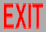# Strings and other things

(an orientation talk on research for incoming graduate students, '03)

# Research topics

• Strings as bound states
• Lorentz covariant quantum spacetime
• New superspaces: x,θ → X(σ),Θ(σ)
• String field theory: Φ[X(σ)]

# Calculability

• Early stages of any theory have little calculability, and consist mostly of existence proofs, hand-waving arguments, order-of-magnitude estimates, etc.
• At some intermediate stage, the theory can be used to determine various constants. Often this is the result of a JWKB expansion, so these constants describe the low-energy behavior of the theory.
• Finally, a more complete description of the theory is found, and functions of energy (& momentum, etc.) can be calculated.

# High-energy predictability

• Nonperturbative Quantum ChromoDynamics is still at the intermediate stage: By methods such as lattices, nonrelativistic quark models, nonlinear σ models, etc., low-energy couplings and masses of the lightest hadrons can be calculated. However, the harmonic-oscillator-like spectrum of particles of arbitrarily high mass (energy) that define confinement, and corresponding scattering amplitudes, can’t. A QCD string would do this. But known strings don’t describe QCD.
• Quantum gravity has perturbative infinities, resulting in a loss of predictability at high (Planck-scale) energies. Superstring theory is perturbatively finite. But the perturbation expansion is inaccurate: It indicates D=10, while nonperturbatively D=11. Nonperturbative effects are only calculable at low energies, bringing us back to square one.

# Strings:

## Is the cure worse than the disease?

• Known strings are in D≥10; reducing D→4 destroys predictability. But renormalizable field theories are already in D≤4.
• Hadrons look like strings. But the known strings don’t describe hadrons.
• Black holes have singularities. Strings don’t seem to address this problem.
• So far, string theory has been a useful toy model, but not practical in describing nature.

# Bound states

• String theory was originally invented to describe hadrons (mesons & baryons). These strings were understood in Quantum ChromoDynamics as composed of partons (quarks & gluons).
• The only explicit string theories found included gravitons. So they were used to describe quantum gravity instead. These were also found to be composed of partons. However, they satisfy the wrong wave equation:
eφ = 0    instead of    ◻φ = 0

# Solutions

• New string theories would be required, of the type already expected for QCD.
• Since QCD is known to exhibit confinement in (at most) D=4, this would correctly predict the (critical) dimension of spacetime, and solve the predictability problem.
• Gravity would become weaker at short distances, exhibiting its constituents, thus eliminating singularities (& black holes).

# Quantum spacetime

More extreme approach to quantum gravity: "Fuzzy” spacetime is quantized because coordinates don’t commute:

[xa,xb] ~ Lab

(This behavior is seen in one model of bound-state gravity.) Make momentum space a hypersphere:
A = (a,5) → LAB = (Lab, La5= xa)

# Supersymmetry

• Predictability at all energies (no “renormalons”) requires finiteness, for both particles and strings. This in turn requires supersymmetry. However, supersymmetry has not yet been observed.
• Superspace introduces fermionic coordinates “θ” to go along with the usual bosonic coordinates “x”. Such formulations are useful for particles, but have problems for strings.